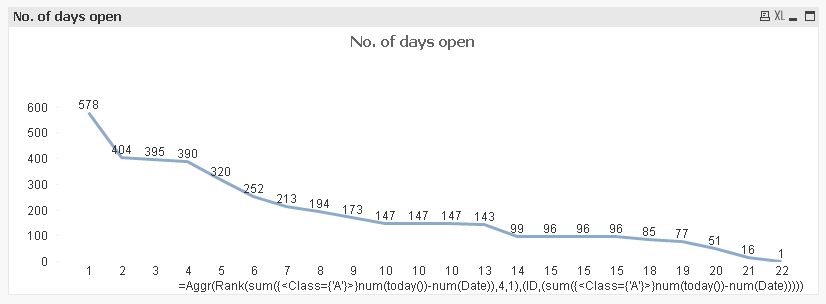QlikView Layout & Visualizations

Discussion Board for collaboration on QlikView Layout & Visualizations.

Announcements
QlikWorld 2020: Join us May 11 - 14, 2020 in Phoenix, AZ. Register early and save \$400. Learn MoreMVP

Re: Sequential number instead of Dimension values

This?Exp (using sortable aggr()):

=Aggr(Rank(sum({<Class={'A'}>}num(today())-num(Date)),4,1),(ID,(sum({<Class={'A'}>}num(today())-num(Date)))))

Contributor II

Re: Sequential number instead of Dimension values

Hi Tresesco,

This is brilliant! Thank you so much!

Just 1 tiny thing - Is it possible to show only distinct values in here? I tried using distinct, but couldn't get it right.Contributor II

Re: Sequential number instead of Dimension values

Or is it possible to number them 10,11,12 instead of 10, 10, 10?MVP

Re: Sequential number instead of Dimension values

Putting Num() around like:

=Aggr(Num(Rank(sum({<Class={'A'}>}num(today())-num(Date)),4)),(ID, (Num(Rank(sum({<Class={'A'}>}num(today())-num(Date)),4)))))

Should have worked, but for some reason (I could not find out yet) few overlapped ranks are not getting sorted that way.

Contributor II

Re: Sequential number instead of Dimension values

Tresesco,

Ok, I will give it a try.

Thank you very much.

Highlighted
Contributor II

Re: Sequential number instead of Dimension values

Hi Tresesco,

This is exactly what I was looking for, it worked perfectly for me. Thank you so much!

=Aggr(Num(Rank(sum({<Class={'A'}>}num(today())-num(Date)),4)),(ID, (Num(Rank(sum({<Class={'A'}>}num(today())-num(Date)),4)))))